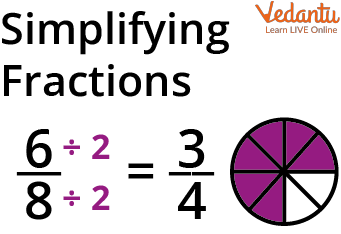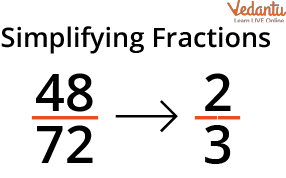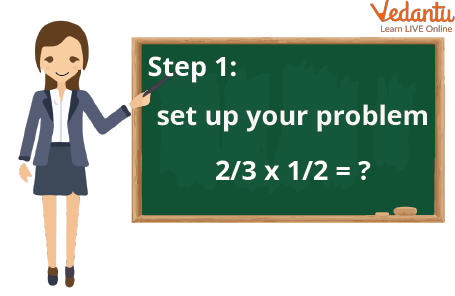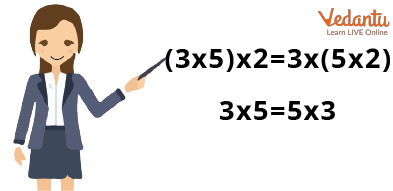Courses
Courses for Kids
Free study material
Free LIVE classes
More

# SimplificationLIVE
Join Vedantu’s FREE Mastercalss

## Introduction to Simplification

In Mathematics, sometimes you get complicated sums and long calculations, which is very common in fraction questions. Some students find it more difficult and take a long time to solve. But don’t worry, this article on simplification in Maths has a resolution for you. It will help you to simplify the fractions in easy ways. In this article, you'll learn what simplification is, how to simplify fractions, and the steps involved in simplifying fractions. You'll also find out how to do it step by step.

## What is Simplification?

As you know, simplification of mathematical problems helps increase scores in exams and is also used in daily life like in malls you get 35% discount, flat discount or 20% discount up to Rs 100. You only need to use fundamental simplification rules to solve equations in this part.

Thus, simplifying an expression implies employing different procedures to reduce it to a simpler version. The operations required to simplify things are done in a set order called BODMAS.

Where,

B = Bracket

0 = of

D = Division

M = Multiplication

S = SubtractionIntroduction to Simplification

To simplify a fraction, reduce it to its most basic form. If a fraction's numerator and denominator only share the number one, it is said to be in its simplest form. The process of simplifying difficulties as we work to tackle fractional problems is essential. The value of the fraction won't change even after we simplify them. This indicates that the true fraction and the simplified fraction are two equivalent fractions. We shall discover a few simple methods for simplifying fractions in this article.Simplifying Fractions

## How to Simplify Fractions?Simplify Fractions

Reducing a fraction to its simplest form is the definition of simplifying a fraction. If a fraction's numerator and denominator are coprime or share only the number one as a common factor, it is said to be in its simplest form. The given fraction is equal to the given fraction in its simplest form. For instance, the fraction $\dfrac{3}{4}$ is in its most basic form since the only element that 3 and 4 have in common is 1.

Let's attempt to gradually simplify the fraction $\dfrac{8}{24}$.

## How to Simplify Fractions Step by Step?How to Simplify Fractions Stepwise

Following is the process of how to simplify fractions step by step -

Step 1: Write the denominator and numerator's factors in step one.

The ratio between 8 and 24 is 1 through 8 factors: 2; 4; and 8

1, 2, 3, 4, 6, 8, 12, and 24 are the 24 factors.

Step 2: Verify the shared factors between the denominator and the numerator. 1, 2, 4, and 8 are the common factors of the numbers 8 and 24.

Step 3: Subtract the common components from the numerator and denominator until there is only one remaining.

The fraction that is so obtained is in its most basic form. Starting with a division of 2, $\dfrac{8}{24}$ becomes $\dfrac{\dfrac{8}{2}}{\dfrac{24}{2}}=\dfrac{4}{12}$.

Up until the point where we can no longer divide by 2, we will keep doing so. Thus, $\dfrac{\dfrac{4}{2}}{\dfrac{12}{2}}=\dfrac{2}{6}$, which is equal to $\dfrac{\dfrac{2}{2}}{\dfrac{6}{2}}=\dfrac{1}{3}$.

The fraction $\dfrac{8}{24}$ is thus represented by its simplest form, $\dfrac{1}{3}$.

Let's return to the original issue of how to make the fraction $\dfrac{8}{24}$ simpler. Between 8 and 24, 8 is their largest common factor. The simplest form of the fraction, which is $\dfrac{1}{3}$, can be obtained by immediately dividing the numerator, 8, by the denominator, 24, and vice versa.

## How to Simplify NumbersHow to Simplify Numbers

Following is the way how to simplify numbers-

• Round it Off.

Produce zeros. 200, therefore, becomes 199.

• Round to an Order of Magnitude.

To the nearest "power of 10," round a lot. The result is that 218 becomes 100 and 2,180 becomes 1,000.

• Slide the Decimal Point.

Rounding zeros are compacted by sliding the decimal point.

This makes 200,000,000 into 2 x 108. (exponential notation).

• Replace it with a Number You Can Recall.

Find a nearby number that you can remember rather than rounding.

Thus, 218 can be easily converted to 222, 210, 200, or any number.

## Solved Examples

Example 1: Simplify: $660 \div 22 \times(2+1)$

Ans: $660 \div 22 \times(2+1)$ (Solve parentheses first)

$=(660 \div 22) \times 3 \text { (From left to right, division appears first) }$

$=30 \times 3=90$

Example 2: Simplify: 37 − [28 + (19 − 7)]

Ans: $37-[28+(19-7)]$ First solve ( )

$=37-[28+12]$ Next, solve []

$=37-40$

$=77$

## Practice Time

Q1. Simplify the expression: $4+(3 \times 4) \div 2$

Ans: 10

Q2. Simplify fractions: (i) $\dfrac{124}{136}$

(ii) $\dfrac{182}{192}$

Ans: (i) $\dfrac{31}{34}$

(ii) $\dfrac{91}96{}$

## Conclusion

Simplification of expressions means rewriting the same algebraic expression with no like terms and in a compact manner. Making something simpler is making it less complex. When you describe a complex mathematical idea to a child in words they can easily understand, you are simplifying.

Last updated date: 22nd Sep 2023
Total views: 110.1k
Views today: 2.10k

## FAQs on Simplification

1. What is the difference between solving and simplifying?

Solving an equation helps determine the value of a variable using real-number properties and combining like terms to make the equation true. Simplifying an algebraic equation implies reducing the expression by employing real-number properties and merging like terms.

2. How to simplify fractions, and what distinguishes solving from simplification?

To simplify fractions, use the instructions listed below:

• Find the numerator and denominator's highest common factor.

• The highest common factor should be used to divide the numerator and denominator.

• The fraction that is so obtained is in its most basic form.

To get the value of the variable in an equation, we must use real number qualities and combine terms that are similar enough to make the equation true. When simplifying an algebraic equation, we need to use real number properties and combine like terms to reduce the statement.

3. How should the simplification of fractions be taught?

Fractions are typically simplified in grades 5 or 6. Use the following guidelines to teach fractions that need to be simplified:

• To help students grasp that 2/4 is equal to 1/2, provide them with the opportunity to work on practical tasks that use rectangular or circular fraction models.

• Give concrete examples of how to simplify fractions.

• Use worksheets for simplifying fractions.